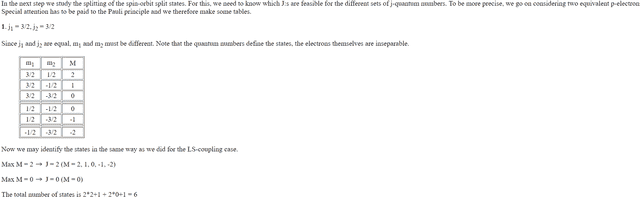# Understanding jj-coupling

proton4ik
Hello everyone! I'm trying to understand how to determine states within the different configuration

## Homework Statement

The question is, why we don't consider Max M=1 -> J=1 while identifying the states for np2 configuration? (http://www.nat.vu.nl/~wimu/JJCoup.html)## The Attempt at a Solution

#### Attachments

Mentor
The question is, why we don't consider Max M=1 -> J=1 while identifying the states for np2 configuration? (http://www.nat.vu.nl/~wimu/JJCoup.html)
Because there is no such state left once those for J=2 have been counted.

There are six possible values of M: 2, 1, 0, 0, -1, -2. The fact that the maximum value of M is 2, there must be a J=2 in there. That J=2 is made up of M=-2,-1,0,1,2, meaning that 5 of those states make up J=2. What you have left is a single state with M=0, hence J=0.

In the hypothetical case where you would have found 9 possible values of M, 2, 1, 1, 0, 0, 0, -1, -1, -2, then after having accounted for J=2, you would be left with
M=1, 0, 0, -1, so now you would have gotten a J=1 as well.

•proton4ik
proton4ik
Because there is no such state left once those for J=2 have been counted.

There are six possible values of M: 2, 1, 0, 0, -1, -2. The fact that the maximum value of M is 2, there must be a J=2 in there. That J=2 is made up of M=-2,-1,0,1,2, meaning that 5 of those states make up J=2. What you have left is a single state with M=0, hence J=0.

In the hypothetical case where you would have found 9 possible values of M, 2, 1, 1, 0, 0, 0, -1, -1, -2, then after having accounted for J=2, you would be left with
M=1, 0, 0, -1, so now you would have gotten a J=1 as well.
Thank you very much for your answer! But still I have a very stupid question left. Why can't values of M for J=2 contain values of M for J=1? What are the properties of M, J that don't allow this?

Mentor
Thank you very much for your answer! But still I have a very stupid question left. Why can't values of M for J=2 contain values of M for J=1? What are the properties of M, J that don't allow this?
I'm not sure I understand your question.

The procedure used here is an accounting procedure, and the books must balance. If you have 6 states and can determine that there is a J=2 component, that component accounts for 5 of the states. You are left with 1 state, which mean you can't have a J=1 component, since that would require an additional 3 states.

More technically, this is a basis transformation: you are going from a basis of states characterised by ##j_1## and ##j_2## and transforming to a basis of states characterised by ##J## and ##M##, and this is done because the latter are eigenstates in the presence of spin-orbit coupling. The two bases must be the same size.